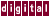# 7.2 Loop Reordering

KAP may find that memory access or other optimizations would be more efficient if an inner loop were moved outside. In these cases, KAP attempts to interchange the loops in the `for` nest to get more efficient operation, as shown in the following example:

```for ( j=0; j<n; j++ )
for ( i=0; i<n; i++ )
a[j][i] = a[j-1][i] + b[j][i];
```

Becomes:

```for ( i = 0; i<n; i++ ) {
for ( j = 0; j<n; j++ ) {
a[j][i] = a[j-1][i] + b[j][i];
}
}
```

See the `/machine` command qualifier.

Previous Page Next Page Contents Index
Command-Line Qualifiers